# PHP Programing language

Operators in PHP
 Previous Home Next

### Operators

Operators are used to perform operations on operands and operators. Operands values of variable in which you can perforn operations and operators are symbols by which you can perform operations on operands.

### Types of Operators:

There are following different types of operators:

1. Arithmetic operators
2. Comparison operators
3. Logical operators
4. Assignment operators
5. String operators
6. Increment/Decrement operators
Arithmetic Operators:
 Operator_Name Symbol Description ```Example (\$A=15, \$B=3)``` Addition + Adds two operands. ```\$A + \$B 15+3=18``` Subtraction - Difference of \$A and \$B. ```\$A - \$B 15-3=12``` Multiplication * Multiply two values. ```\$A * \$B 15*3=45``` Division / Divide numerator by denominator. ```\$A / \$B 15/3=5``` Modulus % Divide A by B and return only the remainder. ```\$A % \$B 15%3=0```

### Example:

```<html>
<head>
<title>Arithmetical Operators</title>
<head>
<body>

<?php
\$A = 50;
\$B = 10;

\$C = \$A + \$B;
echo "Addtion Operator: \$C<br/>";
\$C = \$A - \$B;
echo "\nSubstraction Operator: \$C<br/>";
\$C = \$A * \$B;
echo "\nMultiplication Operator: \$C<br/>";
\$C = \$A / \$B;
echo "\nDivision Operator: \$C<br/>";
\$C = \$A % \$B;
echo "\nModulus Operator: \$C<br/>";
?>
</body>
</html>
```

Output:Comparison Operators:
 Operator_Name Symbol Description ```Example (\$A=15, \$B=3)``` Equal to == Returns true if A equals B. ```A == B 15==3(FALSE)``` Not Equals to != Returns true if A is not equals to B. ```A != B 15!=3(TRUE)``` Less than < Returns true if the value of A is less than the value of B. ```A < B 15 < 3(FALSE)``` greater than > Returns true if the value of A is greater than the value of B. ```A > B 15>3(TRUE)``` Less than or equal to <= Returns true if the value of the A is less than, or equal to, the value of B. ```A <= B 15<=3(FALSE)``` greater than or eual to >= Returns true if the value of A is greater than, or equal to, the value of B. ```A >= B 15>=3(TRUE)``` Identical === Returns true if A equals B in both value and type. ```\$A=100, \$B="100" A === B(FALSE)``` Not Identical !== Returns true if A is not identical to B in both value and type. ```\$A=100, \$B="100" A !==B(TRUE)```

### Example:

```<html>
<head><title>Comparision Operators</title><head>
<body>

<?php
\$A = 15;
\$B = 3;

if( \$A == \$B )
{
echo "== : a is equal to b<br/>";
}
else
{
echo "==: a is not equal to b<br/>";
}
if( \$A != \$B )
{
echo "!= : a is not equal to b<br/>";
}
else
{
echo "!= : a is equal to b<br/>";
}
if( \$A < \$B )
{
echo "< : a is less than  b<br/>";
}
else
{
echo "< : a is not less than b<br/>";
}
if( \$A > \$B )
{
echo "> : a is greater than  b<br/>";
}
else
{
echo "> : a is not greater than b<br/>";
}
if( \$A <= \$B )
{
echo "<= : a is either less than or equal to b<br/>";
}
else
{
echo "<= : a is nieghter less than nor equal to b<br/>";
}

if( \$A >= \$B )
{
echo ">= : a is either grater than or equal to b<br/>";
}
else
{
echo ">= : a is nieghter greater than nor equal to b<br/>";
}

?>
</body>
</html>
```

Output: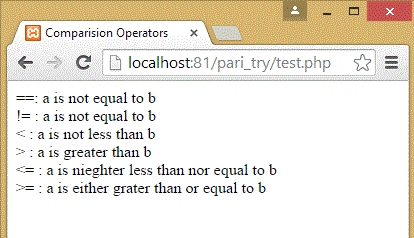Logical Operators:
 Operator_Name Symbol Description Example and && Returns true if A and B are true. ```\$A=6, \$B=6 if(A < 10 && B > 1) returns TRUE``` or || Returns true if either A or B is true. ```\$A=6, \$B=5 (A==5 || B==5) returns TRUE``` xor xor Returns true if either A or B is true, but not both. ```\$A=30, \$B=30 if ((\$A < 25) xor (\$B > 45)) returns FALSE``` not ! Returns true True if a is not true. ```\$A=6 !(\$A) returns FALSE```

### Example:

```<html>
<head><title>Logical Operators</title><head>
<body>

<?php
\$A = 30;
\$B = 29;
if(\$A < 40 && \$B > 25)
{
echo "AND Statement is TRUE<br/>";
}
else
{
echo "AND Statement is False<br/>";
}
if(\$A==40 || \$B==30)
{
echo "OR Statement is TRUE<br/>";
}
else
{
echo "OR Statement is FALSE<br/>";
}
if ((\$A < 25) xor (\$B > 45))
{
echo "XOR Statement is FALSE<br/>";
}
else
{
echo "XOR Statement is TRUE<br/>";
}
if(!(\$A))
{
echo "NOT Statement is FALSE<br/>";
}
else
{
echo "NOT Statement is TRUE<br/>";
}
?>

</body>
</html>
```

Output: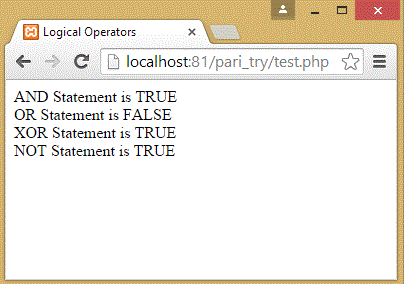Assignment Operators:
 Operator_Name Symbol Description ```Example \$A=20, \$B=30 ``` Assignment = Assigns the right hand value to the left operand. ```\$C = \$A + \$B \$C = 20+30 \$C = 50``` Addition Assignment += Adds two numbers and assigns the result to the first. ```\$C += \$A \$C = \$C + \$A \$C = 50+20 = 70``` Subtraction Assignment -= Subtract two numbers and assigns the result to the first. ```\$C -= \$A \$C = \$C - \$A \$C = 70-20 = 50``` Multiplication Assignment *= Multiply two numbers and assigns the result to the first. ```\$C *= \$A \$C = \$C * \$A \$C = 50*20 = 1000``` Division Assignment /= Divide two numbers and assigns the result to the first. ```\$C /= \$A \$C = \$C / \$A \$C = 1000/20 = 50``` Modulus Assignment %= Modulus of two numbers and assigns the result to the first. ```\$C %= \$A \$C = \$C % \$A \$C = 50%20 = 10```

### Example:

```<html>
<head><title>Assignment Operators</title><head>
<body>

<?php
\$A = 20;
\$B = 30;

\$C = \$A + \$B;  //Assign the value of addition to \$C
echo " '='  Operator Value: \$C <br/>";

\$C += \$A;  //Value of C is 50+20=70
echo " '+='  Operator Value: \$C <br/>";

\$C -= \$A;  //Value of C is 70-20=50
echo " '-='  Operator Value: \$C <br/>";

\$C *= \$A;  //Value of C is 50*20=1000
echo " '*='  Operator Value: \$C <br/>";

\$C /= \$A;  //Value of C is 1000/20=50
echo " '/='  Operator Value: \$C <br/>";

\$C %= \$A;  //Value of C is 50%20=10
echo " '%='  Operator Value: \$C <br/>";
?>

</body>
</html>
```

Output: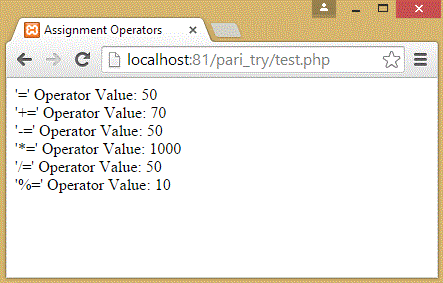String Operators:
 Operator_Name Symbol Description Example Concatenation Operator . Combines two string variables. ```\$A = "Good"; \$B = "Morning"; \$C = "India"; \$str = \$A . \$B . \$C; echo \$str;``` Concatenating Assignment Operator .= Combines two string variables and assigns the result to the first. ```\$A = "Hello"; \$A .= "World"; echo \$A; ```

### Example:

```<html>
<head><title>String Operators</title><head>
<body>

<?php
\$A = "Good ";
\$B = "Morning ";
\$C = "India";

echo "Sting Concatenation Operator (.):  ";
\$str = \$A . \$B . \$C;
echo \$str;

echo "<br/>String Concatenating Assignment Operator (.=):  ";
\$A .= \$B;
echo \$A;

?>
</body>
</html>
```

Output: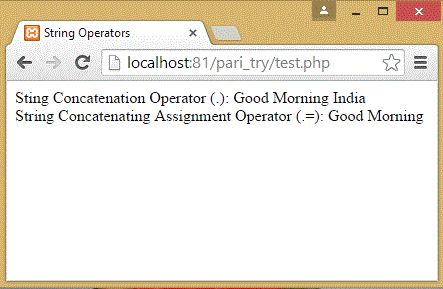Increment/Decrement Operators:
 Operator_Name Symbol Description ```Example \$A=20, \$B=30 ``` Increment Operator ```Pre(++\$A) Post(\$A++) ``` Increases the value of variable by 1. ```Pre: \$B = 5; \$A = ++\$B; \$A = 6, \$B = 6 Post: \$B = 5; \$A = \$B++; \$A = 5, \$B = 6 ``` Decrement Operator ```Pre(--\$A) Post(\$A--)``` Decreases the value of variable by 1. ```Pre: \$B = 6; \$A = --\$B; \$A = 5, \$B = 5; Post: \$B = 5; \$A = \$B--; \$A = 5; \$B = 4; ```

### Example:

```<html>
<head><title>Increment/Decrement Operators</title><head>
<body>

<?php
\$A = 10;
echo "Value of A is : \$A";
echo "<br/>After Pre-increment value of A ( i.e. ++\$A ) is: ".++\$A;
echo "<br/>Value of A is : \$A";
echo "<br/>After Post-increment value of A ( i.e. \$A++ ) is: ".\$A++;
echo "<br/>Value of A is : \$A";
echo "<br/>After Pre-decrement value of A ( i.e. --\$A ) is: ".--\$A;
echo "<br/>Value of \$A is : \$A";
echo "<br/>After Post-decrement value of A ( i.e. \$A-- ) is: ".\$A--;
?>

</body>
</html>
```

Output: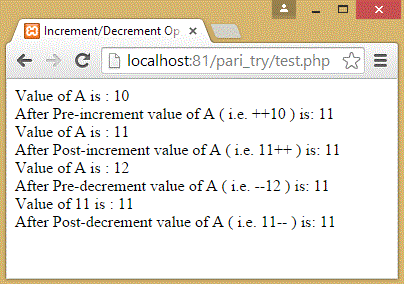Previous Home Next